# Electronics and Communication Engineering - Automatic Control Systems - Discussion

1.

For the system in the given figure the characteristic equation is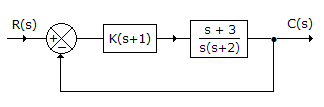[A].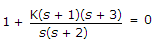[B].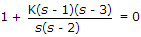[C]. K(s + 1)(s + 3) = 0 [D]. s(s + 2) = 0

Explanation:

Transfer function =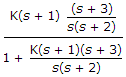.

Hence characteristic equation is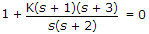.

 Shahwaz said: (May 6, 2014) Where is output and input of this system?

 Ramya said: (May 29, 2015) Here characteristics equation is 1+G(s)H(s) = 0.

 Sujji said: (Nov 4, 2015) For unity feedback systems the transfer function is G (S)/1+G (S). So the characteristic equation is 1+G (S) = 0.

 Navtej Bhangu said: (May 26, 2016) Characteristic equation is given by 1 + G(S) H(S) = 0. So, H(s) = 0.

 Sagar Kumar said: (Jun 29, 2016) This is helpful to improve the technical skills. Please provide more advance questions.

 Shristy said: (May 14, 2017) I think the H(s) is not 0 it is 1.

 Abhishek said: (Sep 4, 2017) I think k(s+1) (s+3) =0 will be the answer.

 Chanchal said: (Feb 2, 2018) @ALL. As per the question, characteristics eq. 1+ G(s).H(s). Here H(s) is unity. So option A is right.

 Mufeed Ardouno said: (May 1, 2019) Thank you all.

 Mufeed said: (May 1, 2019) For unity feedback systems the transfer function is G (S)/1+G (S). So, the characteristic equation is 1+G (S) = 0.